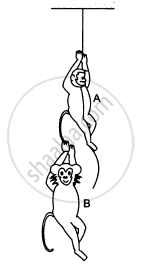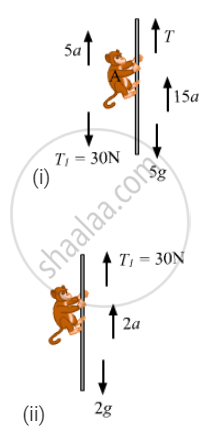# The Monkey B, Shown in the Figure (5−E20), is Holding on to the Tail of Monkey a that is Climbing up a Rope. the Masses of Monkeys a and B Are 5 Kg and 2 Kg, Respectively. If a Can Tolerate a - Physics

Sum

The monkey B, shown in the following figure, is holding on to the tail of monkey A that is climbing up a rope. The masses of monkeys A and B are 5 kg and 2 kg, respectively. If A can tolerate a tension of 30 N in its tail, what force should it apply on the rope in order to carry monkey B with it? Take g = 10 m/s2.#### Solution

Let the acceleration of monkey A upwards be a, so that a maximum tension of 30 N is produced in its tail.T − 5g − 30 − 5a = 0    ...(i)
30 − 2g − 2a = 0    ...(ii)
From equations (i) and (ii), we have:
T = 105 N   (max.)
and a = 5 m/s2
So, A can apply a maximum force of 105 N on the rope to carry monkey B with it.
For minimum force, there is no acceleration of A and B.
T1 = weight of monkey B
⇒ T1 = 20 N
Rewriting equation (i) for monkey A, we get:
T − 5g − 20 = 0
⇒ T = 70 N
∴ To carry monkey B with it, monkey A should apply a force of magnitude between 70 N and 105 N.

Concept: Newton’s Second Law of Motion
Is there an error in this question or solution?

#### APPEARS IN

HC Verma Class 11, Class 12 Concepts of Physics Vol. 1
Chapter 5 Newton's Laws of Motion
Q 38 | Page 82